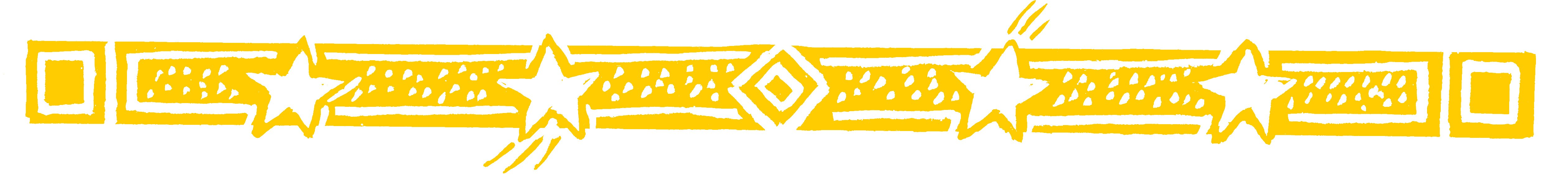Teacher - show your class the easy way to add and subtract fractions.

Use VERTICALLY AND CROSSWISE to write the answer straight down!

•2 x 5 = 10 and 1 x 3 = 3. Then 10 + 3 = 13.
The bottom of the fraction is just 3 x 5 = 15.
You multiply the bottom numbers together.

So:

•Subtracting is just as easy: multiply crosswise as before, but then subtract:

•Question Comment !
 Tutorial Title Question Title No Questions 0 Questions Asked 0 Skipped Questions 0 Correctly Answered 0 Wrongly Answered 0 Total Question Attempts 0 Current Question Attempts 0

Skip Question

Reset Test

Halt Test

 No Questions 0 Questions Asked 0 Skipped Questions 0 Correctly Answered 0 Wrongly Answered 0 Total Question Attempts 0

Time Taken

CloseShow Your Class... nature is the
realisation of the
simplest conceivable
mathematical ideas
EINSTEIN